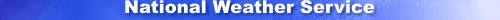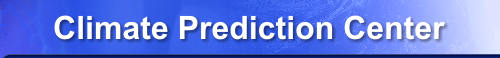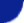Site Map News Organization SearchHOME > Monitoring_and_Data > Oceanic and Atmospheric Data > Reanalysis: Atmospheric Data > wgrib2-new_grid_ipipt

Introduction

The -new_grid option interpolates from one grid into another. The -new_grid_interpolation option selects the interpolation method. The -new_grid_ipopt option modifies the parameters used by the interpolation method. The -new_grid_ipopt option MUST FOLLOW the -new_grid_interpolation option because the latter will overwrite the IPOPT values with the default values for that interpolation method.

1. bilinear: ipopt is not used
2. bicubic:
1. ipopt(1) = 0: straight bicubic
2. ipopt(1) = 1: constrained to range of 4 neighboring points
3. ipopt(2) = %data converage for output point, default=50%
3. nearest neighbor: ipopt is not used
4. budget: ipopt is not used
5. spectral: (alpha)
1. ipopt(1) = 0: triangular truncation
2. ipopt(1) = 1: rhomboidal truncation
3. ipopt(2) = N: truncation number
4. ipopt(2) = -1: sensible truncation number, based on grid (default for now, will be changed)
note: should specify these values by -new_grid_interpolation

when ipopt(2) == -1, sensible trunction number
IROMB = ipopt(1)
IDRT=4 FOR GAUSSIAN GRID
IDRT=0 FOR EQUALLY-SPACED GRID INCLUDING POLES
IDRT=256 FOR EQUALLY-SPACED GRID EXCLUDING POLES

IF(IROMB.EQ.0.AND.IDRTI.EQ.4) MAXWV=(JMAXI-1)
IF(IROMB.EQ.1.AND.IDRTI.EQ.4) MAXWV=(JMAXI-1)/2
IF(IROMB.EQ.0.AND.IDRTI.EQ.0) MAXWV=(JMAXI-3)/2
IF(IROMB.EQ.1.AND.IDRTI.EQ.0) MAXWV=(JMAXI-3)/4
IF(IROMB.EQ.0.AND.IDRTI.EQ.256) MAXWV=(JMAXI-1)/2
IF(IROMB.EQ.1.AND.IDRTI.EQ.256) MAXWV=(JMAXI-1)/4

Note: The values are appropriate for the spectral GFS Gaussian grid
because the grid values were derived from the spherical harmonics.
The values are not appropriate for the FV3 GFS Gaussian grid
because the grid values were derived by a bilinear interpolation
(mostly) from the cubed sphere grid.

Since NCEP is transitioning to a FV3 model, the grid point values
are not the exact representation of the model fields but rather
a bilinear interpolation from the cubed sphere.  So a high-wavenumber
noise is being added to the grid values.  So the appropriate spectral
representation will have filter applied to the spectral represenation.
The math hasn't been worked out.

6. neighbor-budget: not enabled by wgrib2

Usage

-new_grid_ipopt X
X = integer or integer:integer